Select Page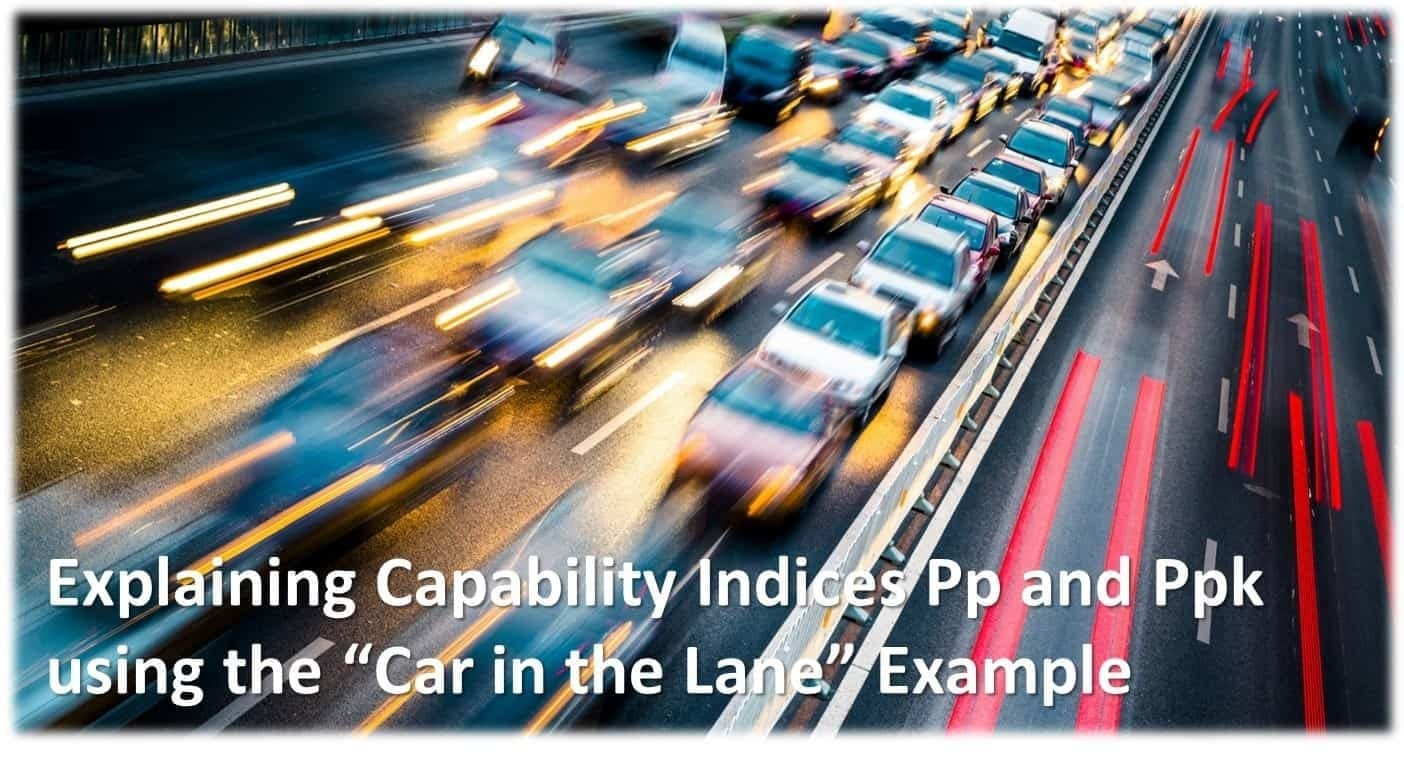## How Traffic and Process Capability (Ppk) Relate?

As a teacher and a mentor, i am constantly looking for new ways to make complex subjects less complex using games, simulations stories, analogies., etc. My colleagues and I have found that the topic of Process Capability and how to interpret the Long Term and Short Term capability indices tend to make our students “eyes glaze over”.

What we came up with is a spin on other examples that i found on the web. We use an analogy of a car in a lane of a highway. The lane is bordered on both sides by concrete barriers. Cars are 60+ miles per hour in this lane.

Before we go into the analogy, we must first define what the CAR and the LANE represent.

• The Width of the CAR is the Voice of the Process (VOP).
• The Width of the Car (or VOP) is always equal to six standard deviations.wide.
• VOP is a dynamic metric because as we add more data to the data set the standard deviation changes
• The Width of the Lane (or VOC)
• The Width of the Lane (or VOC) is also called the Tolerance because this is what the customer will tolerate
• VOC is a static metric meaning that it only changes as the customer changes it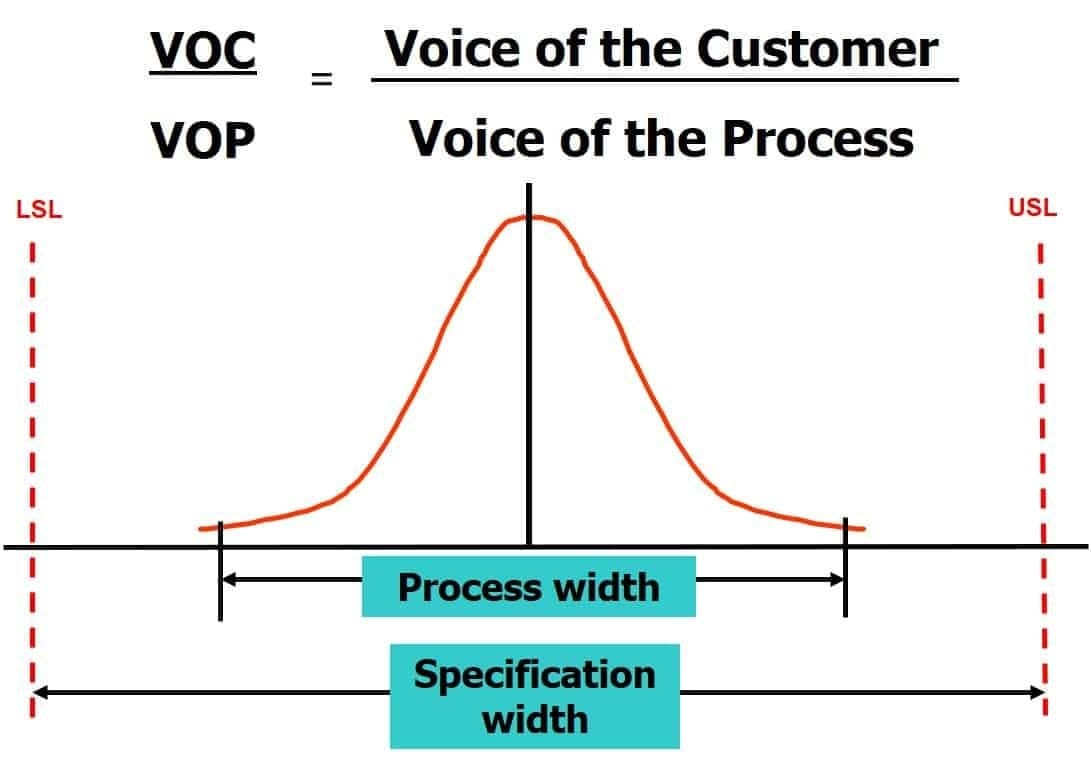Now that we have a better understanding of how the analogy of the CAR and the LANE relate to VOP and VOC, we can use the CAR and the LANE example to explain the Process Capability Indices Ppk.

We only explain the Capability Indices Ppk because it is considered “Real Capability” and will give you truer representation of you processes capability to meet the requirement set by the customer.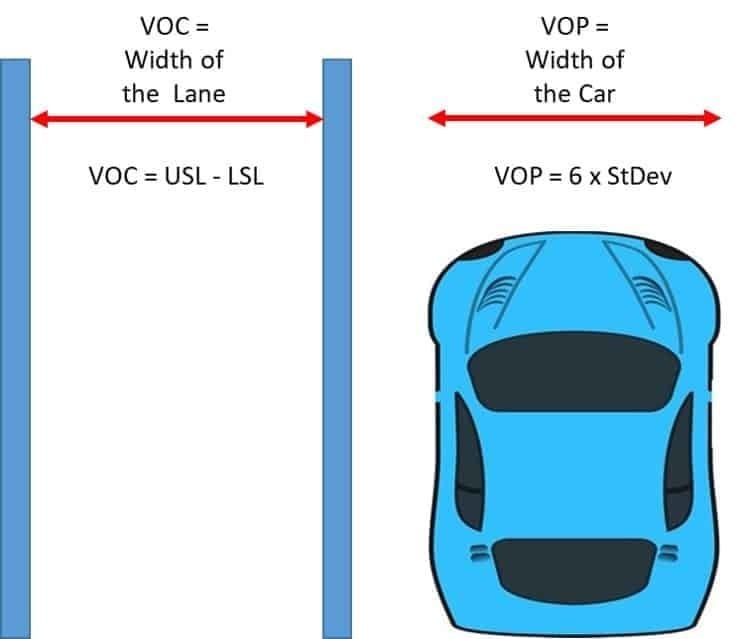Scenario:

You are in a city that has a road with one of two lanes under construction.

The are concrete barriers on both sides of the single lane.

Cars are going 60 MPH or more in this single lane

#### The Capability Indices “Pp” Compares the VOP (Car) to the VOC (Lane)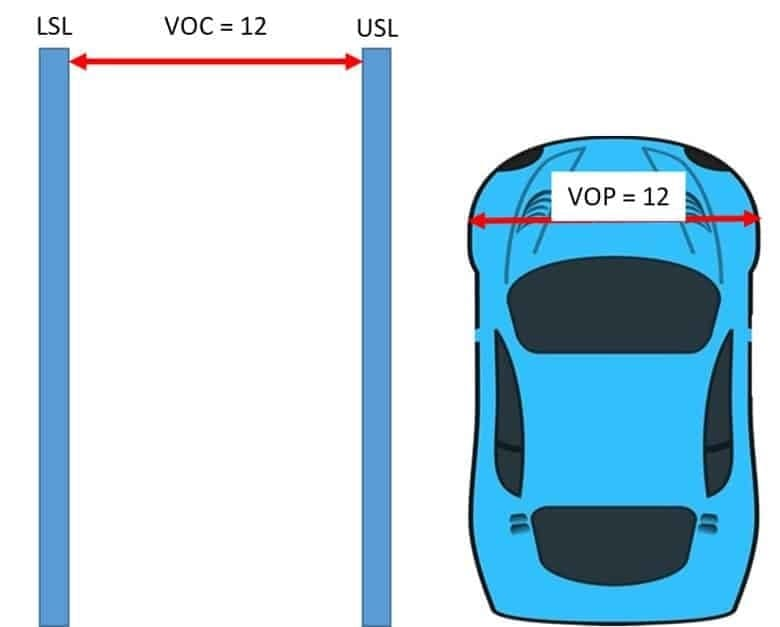The Calculation for Pp = VOC / VOP

In this example Pp = 12/12 = 1

The Calculation for VOC = USL – LSL

The Calculation for VOP = 6 x StDev

“Pp” does not care whether the car is in the lane. It just compares the size of the lane to the size of the car

#### The Capability Indices “Ppk” not only Compares the VOP to the VOC but also tell you where the car is in the lane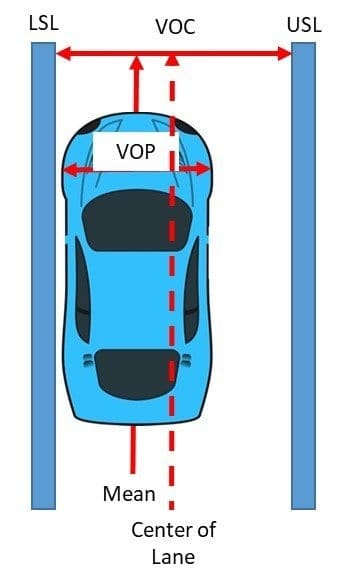Ppk is the Minimum of two Calculations Ppu (Pp Upper) and Ppl (Pp Lower)

The Minimum of the two calculations tell us what side of the Center of Lane the is the Center of the Car

The Calculation for Ppk = Min (Ppu, Ppl)

• Ppu = (USL – Mean) / (3 x StDev)
• Ppl = (Mean – LSL) / (3 x StDev )

Ppk = Min of Ppu and Ppl

#### Can Ppk be less than 1?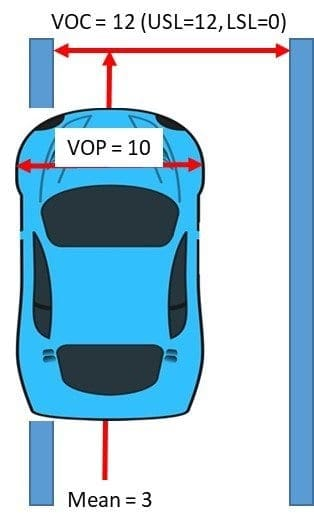The Calculation for Ppk = Min (Ppu, Ppl)

• Ppu = (USL – Mean) / (3 x StDev) (in this example: (12 – 3) / 5 = 1.80)
• Ppl = (Mean – LSL) / (3 x StDev) (in this example: (3 – 0) / 5 = 0.6)
•

Ppk = Ppl (in this example: 0.6)

If the side of the car exceeds the lane then the Ppk is less than 1

If the center of the car has not exceeded the lane but the side of the car has then the Ppk is less than 1 and greater than 0

#### Can Ppk be 0 (Zero)?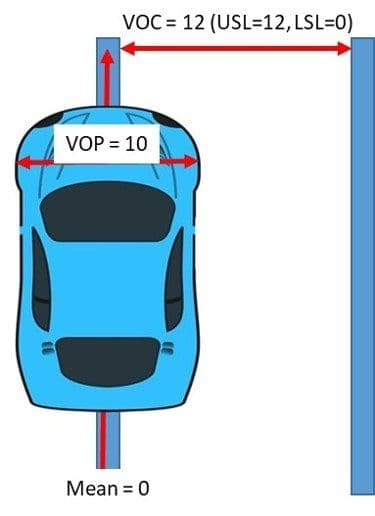The Calculation for Ppk = Min (Ppu, Ppl)

• Ppu = (USL – Mean) / (3 x StDev) (in this example: (12 – 0 )/ 5 = 2.40)
• Ppl = (Mean – LSL) / (3 x StDev) (in this example: (0 – 0) / 5 = 0.0)

Ppk = Ppl (in this example: 0.0)

If the middle of the car equals the lane then the Ppk is 0 (Zero)

#### Can Ppk be less than 0?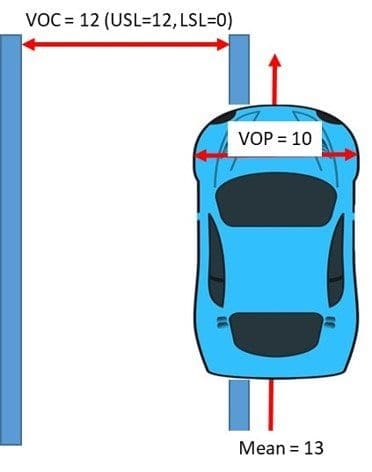The Calculation for Ppk = Min (Ppu, Ppl)

• Ppu = (USL – Mean) / (3 x StDev) (in this example: (12 – 13) / 5 = -0.2)
• Ppl = Mean – LSL / 3 x StDev (in this example: (13 – 0 )/ 5 = 2.6)

Ppk = Ppu (in this example: -0.2)

If the middle of the car exceeds the lane then the Ppk is less than 0 (zero) or negative

#### What if the Car is in the Middle of the Lane?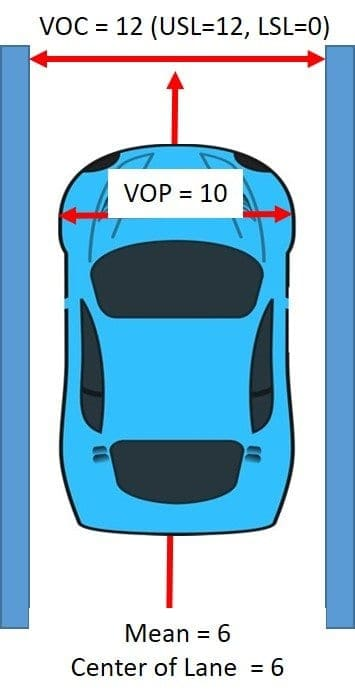The Calculation for Ppk = Min (Ppu, Ppl)

• Ppu = (USL – Mean) / (3 x StDev) (in this example: (12 – 6) / 5 = 1.2)
• Ppl = (Mean – LSL) / (3 x StDev) (in this example: (6 – 0) / 5 = 1.2)

Ppk = Ppu (in this example: 1.2)

The Calculation for Pp = VOC / VOP (In this example Pp = 12/10 = 1.2)

If the middle of my car equals the middle of the lane, then Pp, Ppu, Ppl and Ppk are the same

Feel free to use this analogy to teach others the concept of the Process Capability Indices Ppk. If this article has helped you or your colleagues to better understand these concepts please share with us in the comments below.LEARN THE 10 SIMPLE STEPS THAT WE TEACH LEAN SIX SIGMA GREEN BELTS TO GET AMAZING RESULTS FROM THEIR PROJECTS.